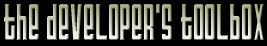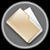This section of the archives stores flipcode's complete Developer Toolbox collection, featuring a variety of mini-articles and source code contributions from our readers.Simple Memory Manager   Submitted byEditor's Note: The description can be found in the code's comments.

 ```/*Editor's note: COTD Entry: A simple memory manager by Juan Carlos Arevalo Baeza [jcab@roningames.com] I'd like to share a piece of code I use quite a bit. It is a fully functional, double-headed frame-based memory manager. If you need a detailed explanation of what this is, you can check in the book "Game Programming Gems", page 92, where there's a nice article about it. In here, I'll just describe the general concept, and a few of the features that this manager has. Basically, a frame-based memory manager is a manager where the heap acts as a stack: allocating and deallocating are as simple as moving the top of the stack. This has a very apparent benefit: it's very fast. It also has a very apparent caveat: allocations and deallocations must be perfectly nested, or else the heap can get corrupted. The heap manager I'm posting here has the additional benefit that it uses two stacks: one growing from the bottom up and another one growing from the top down. Those heaps are totally independent, except for the fact that, at any given moment, the total amount of memory allocated in both heaps must not be greater than the size of the buffer reserved for the heap. So, the heap is full when the two tops-of-the-stacks meet somewhere in the buffer. I've cleaned the code, added a couple extra comments, and made a little example function, showing how it's used. */// Assert helpers. #pragma warning (4: 4390) // empty controlled statement found; is this the intent? #ifndef NDEBUG // DoAssert() is defined elsewhere. Use your favorite assert macro or function. bool DoAssert(bool c, const char *cstr, const char *file, unsigned int line); #define ASSERT(c) if (!DoAssert(c, #c, __FILE__, __LINE__)) #define VERIFY(c) ASSERT(c) #else #define ASSERT(c) if (false) // ASSERT disappears in release builds #define VERIFY(c) if (!(c)) // VERIFY still executes the condition #endif // Forward helper class declarations. Need them here because they'll be made friends. class SaveHeapLowState; class SaveHeapHighState; // Heap manager class. class SimpleHeap { // Granularity (and alignment) values. enum { GRAN_BITS = 2, // Granularity bits. 2 bits = 4 bytes, 3 bits = 8 bytes, and so on. GRAN_NUM = 1 << GRAN_BITS, // Granularity bytes. See above. GRAN_MASK = GRAN_NUM - 1, // Mask value for granularity adjustments. }; // Data. unsigned int m_Size; // Total size of the heap. char * m_MemAlloc; // Allocated memory pointer. char * m_Data; // Data pointer. It can differ from the allocated pointer if there is an alignment adjustment to it. unsigned int m_LowEnd; // Limit position of the low heap. unsigned int m_HighEnd; // Limit position of the high heap. public: // Accumulated statistics. unsigned int m_LowMax; // Maximum memory ever allocated by the low heap. unsigned int m_HighMax; // Maximum memory ever allocated by the high heap. unsigned int m_TotalMax; // Maximum memory ever allocated by the both heaps simultaneously. // Constructor/destructor. __forceinline SimpleHeap(unsigned int size = 256*1024): m_Size(size), m_Data(NULL), m_LowEnd(0), m_HighEnd(size), m_LowMax(0), m_HighMax(0), m_TotalMax(0) { ASSERT(m_Size > 0); m_Size = (m_Size + GRAN_MASK) & ~GRAN_MASK; m_MemAlloc = new char[m_Size + GRAN_MASK]; m_Data = (char *) (((long) m_MemAlloc + GRAN_MASK) & ~GRAN_MASK); } __forceinline ~SimpleHeap() { ASSERT(m_MemAlloc != NULL); delete[] m_MemAlloc; } // Utility management functions. __forceinline void Reset() { LowReset(); HighReset(); } __forceinline unsigned int GetTotalMem() { return m_Size; } __forceinline unsigned int GetFreeMem() { return m_HighEnd - m_LowEnd; } __forceinline unsigned int GetAllocMem() { return m_Size - m_HighEnd + m_LowEnd; } // Low heap. friend class SaveHeapLowState; __forceinline unsigned int GetLowAllocMem() { return m_LowEnd; } __forceinline void *LowAlloc(unsigned int size) { ASSERT(m_Data != NULL) return NULL; VERIFY(size > 0) return NULL; size = (size + GRAN_MASK) & ~GRAN_MASK; VERIFY(m_LowEnd + size <= m_HighEnd) return NULL; void *r = m_Data + m_LowEnd; m_LowEnd += size; if (m_LowMax < m_LowEnd) { m_LowMax = m_LowEnd; } if (m_TotalMax < m_Size - m_HighEnd + m_LowEnd) { m_TotalMax = m_Size - m_HighEnd + m_LowEnd; } return r; } template < class T > __forceinline T *LowAlloc(T *&t) { return t = (T *) LowAlloc(sizeof(T)); } template < class T > __forceinline T *LowAlloc(T *&t, unsigned int n) { return t = (T *) LowAlloc(sizeof(T) * n); } __forceinline void LowReset() { m_LowEnd = 0; } // High heap. friend class SaveHeapHighState; __forceinline unsigned int GetHighAllocMem() { return m_Size - m_HighEnd; } __forceinline void *HighAlloc(unsigned int size) { ASSERT(m_Data != NULL) return NULL; VERIFY(size > 0) return NULL; size = (size + GRAN_MASK) & ~GRAN_MASK; VERIFY(m_LowEnd <= m_HighEnd - size) return NULL; m_HighEnd -= size; if (m_HighMax < m_Size - m_HighEnd) { m_HighMax = m_Size - m_HighEnd; } if (m_TotalMax < m_Size - m_HighEnd + m_LowEnd) { m_TotalMax = m_Size - m_HighEnd + m_LowEnd; } return m_Data + m_HighEnd; } template < class T > __forceinline T *HighAlloc(T *&t) { return t = (T *) HighAlloc(sizeof(T)); } template < class T > __forceinline T *HighAlloc(T *&t, unsigned int n) { return t = (T *) HighAlloc(sizeof(T) * n); } __forceinline void HighReset() { m_HighEnd = m_Size; } }; // These classes are used to save and restore the state. class SaveHeapLowState { SimpleHeap &m_Heap; unsigned int m_State; public: SaveHeapLowState(SimpleHeap &heap): m_Heap(heap), m_State(heap.m_LowEnd) {} ~SaveHeapLowState() { m_Heap.m_LowEnd = m_State; } }; class SaveHeapHighState { SimpleHeap &m_Heap; unsigned int m_State; public: SaveHeapHighState(SimpleHeap &heap): m_Heap(heap), m_State(heap.m_HighEnd) {} ~SaveHeapHighState() { m_Heap.m_HighEnd = m_State; } }; // These helper functions are used to allocate arrays of a specified type. template < class T > T *LowAlloc(SimpleHeap &heap, unsigned int num = 1) { return (T*)heap.LowAlloc(num); } template < class T > T *HighAlloc(SimpleHeap &heap, unsigned int num = 1) { return (T*)heap.HighAlloc(num); } Of special interest here are the classes SaveHeapLowState and SaveHeapHighState. Using them to handle the deallocations makes them potentially much more safe. Also, there are a couple of template functions that help with allocating arrays. As an added feature, the heap keeps track of how much memory has been used by each heap, and by both simultaneously. An example of how the memory manager could used:void func() { SimpleHeap heap(1024*1024); // Reserve one megabyte. { SaveHeapLowState lowstate(heap); // Save the current state of the low heap. { int **pintarray; heap.LowAlloc(pintarray, 50); int i; for (i = 0; i < 50; ++i) { SaveHeapHighState highstate(heap); // Save the current state of the high heap. int *tempbuf = LowAlloc(heap, i); // using the helper function. bool error = false; heap.LowAlloc(pintarray[i], 10); // Here, you would fill in the pintarray[i] array with an algorithm that uses the tempbuf. if (error) { // Everything is automatically deallocated here by the destructors, in the appropriate order. return; } // The high heap is reset at this point, thanks to the destructor of SaveHeapHighState. } // Here, you have the arrays inside of pintarray filled in with values. } // The low heap is reset at this point, thanx to the destructor of SaveHeapLowState. } // The heap is empty here. All the memory is available. // The heap is released by its destructor at this point. } Note that, in order to compile this code as-is, you need MSVC++ 6.0. If you're using another compiler, you should add at the beginning:#define __forceinline inline A last note: I decided to leave the asserts in place, so I included a simplified version of the definition of the ASSERT and VERIFY macros we use. The only thing that's missing is the DoAssert() function. You can use something like:bool DoAssert(bool c, const char *cstr, const char *file, unsigned int line) { if (!c) { fprintf(stderr, "%s(%d): ASSERT FAILED!! %s\n", file, line, cstr); } return c; } ```